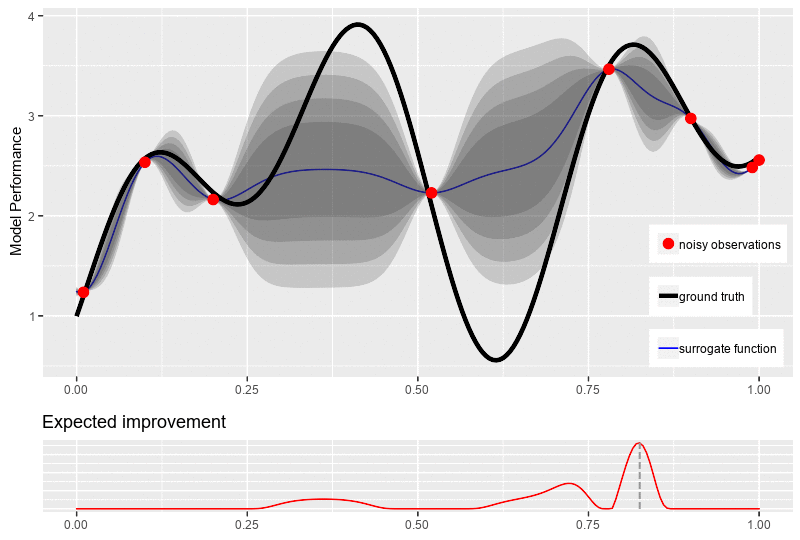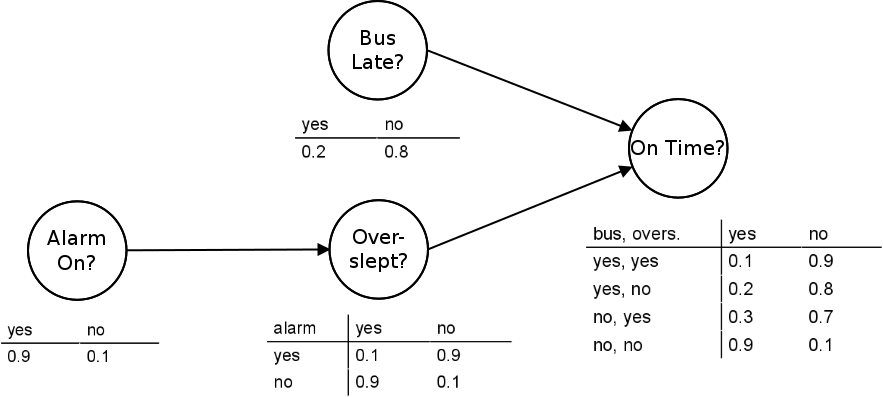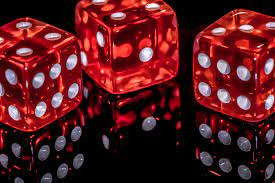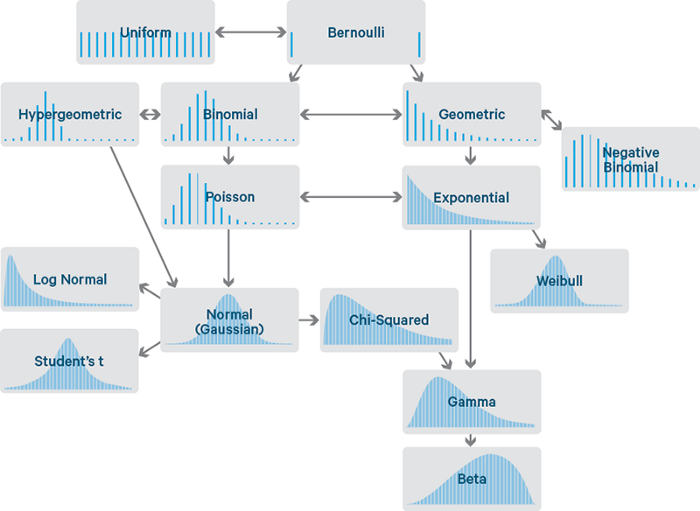## Bayesian OptimizationDecember 10th, 2022

A brief introduction of Bayesian optimization for black-box optimization with Gaussian Process and acquisition functions.

## Bayesian NetworkNovember 29th, 2022

A gentle introduction to Bayesian Network.

## Introduction to Probability - Part 2September 28th, 2022

Mathematic concepts for probabilistic Machine Learning.

## Introduction to Probability - Part 1September 8th, 2022

Introduction to Statistic, Probability, and Distributions.